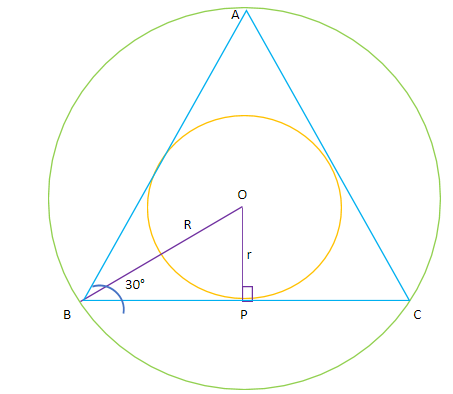QuestionAnswers

# The circumference of a circle circumscribing an equilateral triangle is $24\pi$units. Find the area of the circle inscribed in the equilateral triangle.

Hint: We will first draw the figure and then we will first calculate the radius of the circle circumscribing the equilateral triangle. Then, we will construct a perpendicular arm from the centre of the incircle to the base of the triangle. Since, the triangle is an equilateral triangle, the bisector will part it equally into two. Using the sine function as sin$\theta$= $\dfrac{{perpendicular}}{{hypotenuse}}$, we will calculate the value of radius of the incircle. Once we get the value of the radius of the incircle, we can calculate the area by using the formula of area of the circle: A = $\pi {r^2}$.

Let us draw the figure:Here, green circle indicates the circumcircle with radius R and orange circle indicates the incircle with the radius r.
We construct a perpendicular arm OP at the base BC of the triangle ABC. OB is the angle bisector of the $\angle ABC$ and as it is an equilateral triangle so every angle must be equal to 60°. Therefore, the angle OBP will be 30°.
Let us first calculate the radius of the circumcircle. The circumference of a circle is given by 2$\pi$r. let R be the radius of the circumcircle and its circumference is given as $24\pi$units.
Therefore, 2$\pi$R = $24\pi$
$\Rightarrow$2R = 24
$\Rightarrow$R= 12 units.
Now, for the radius r of the incircle: in triangle ABC,
Sin B = $\dfrac{{perpendicular}}{{hypotenuse}}$
$\Rightarrow$$\sin {30^ \circ } = \dfrac{r}{R}$
$\Rightarrow \dfrac{1}{2} = \dfrac{r}{R} \\ \Rightarrow r = \dfrac{R}{2} \\ \Rightarrow r = \dfrac{{12}}{2} = 6 \\$
Therefore, the radius of the incircle is found to be 6 units.
Now, we can calculate the area of incircle using the formula of the area of the circle: $\pi {r^2}$
$\Rightarrow area(incircle) = \pi {r^2} = \pi \times {(6)^2} \\ \Rightarrow area(incircle) = 36\pi \\$
Hence, the area of the circle inscribed in the equilateral triangle is 36 $\pi {units}^2$.

Note: In such questions, you may get confused with the language of the questions. So, it is advisable that you first draw the figure of the question. You may face difficulty while calculating the radius of the incircle because we are required to do a bit of construction.